Next: Multiple sequence alignment. Up: Definitions Previous: Dayhoff matrices.

#### Sequence alignment.

Aligning sequences is the process of associating some positions of each sequence with a position of the other sequence. This association preserves the order of the sequences. E.g.
 VNRLQQNIVSL____________EVDHKVANYKPQVEPFGHGPIFMATALVPGLYLGVPWF
VNRLQQSIVSLRDAFNDGTKLLEELDHRVLNYKPQANPFGNGPIFMVTAIVPGLHLGAPWF

Unassociated positions are called insertions (or their counterpart deletions). Aligning protein sequences by dynamic programming (DP) using Dayhoff matrices is equivalent to finding the alignment which maximizes the probability that the two sequences evolved from an ancestral sequence as opposed to being random sequences. More precisely, we are comparing two events

a)
that the two sequences are independent of each other, and hence an arbitrary position with amino acid i aligned to another arbitrary position with amino acid j has the probability equal to the product of the individual frequencies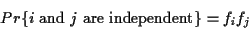b)
that the two sequences have evolved from some common ancestral sequence after t units of evolution.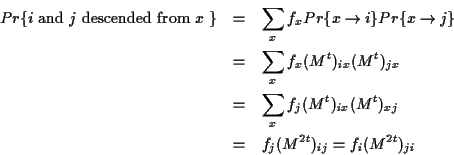We use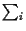as a shorthand for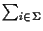, that is a sum over all symbols of the alphabet. The entries of the Dayhoff matrix are the logarithm of the quotient of these two probabilities.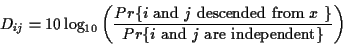Since DP maximizes the sum of the similarity measure, DP maximizes the sum of the logarithms or maximizes the product of these quotients of probabilities. As a conclusion, DP finds the alignment which maximizes the probability of having evolved from a common ancestor (a maximum likelihood alignment) against the null hypothesis of being independent. This makes aligning sequences using Dayhoff matrices a soundly based algorithm.Next: Multiple sequence alignment. Up: Definitions Previous: Dayhoff matrices.
Gaston Gonnet
1998-07-14